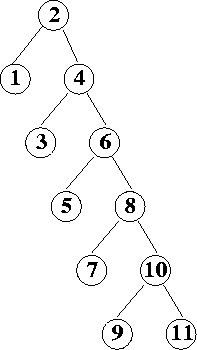CSE 5311 Fall 1999

Quiz #3

1.
(6 points.) Demonstrate two consecutive tree rotations that will reduce the height of the binary tree below from 5 to 3.2.
(8 points.) Draw the 11-slot hash table (m = 11) that results from using the primary hash function h(i) = (2i+5) mod 11 to hash keys 12, 44, 13, 88, 23, 94, 11, 39, 20, 16, and 5. Handle collisions by double hashing, using h'(i) = 7 - (i mod 7) as a secondary hash function.

3.
(6 points.) Binary heaps and hash tables are two well-known data structures for maintaining dynamic sets of elements. Describe an application scenario where you should use heaps instead of hash tables, and another scenario where you should use hash tables instead of heaps.